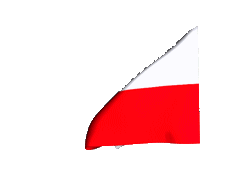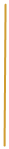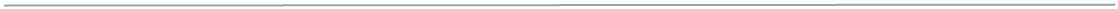Middle School
Math Curriculum

1) Natural Numbers
A. Playing with numbers;
I.   Finding missing numbers by using addition, subtraction, multiplication
and division,
II.  Finding missing numbers based on the properties of numbers,
III. Solving arithmetic problems using order of operation.
B. Divisibility of numbers;
I.   Checking and showing divisibility of numbers,
II.  Finding the divisor and a multiple based on properties of numbers.
C. Exponents;
I.   Properties of exponents,
II.  Finding ones in some exponents.
2) Fractions and decimals
A. Using properties of decimal number system to create decimals;
I.   Converting fraction to decimal and decimal to fraction,
II.  Conversion of units: weight, length.
3) Acting on fractions
A. Solving problems on fractions and decimals using addition, subtraction,
multiplication and division;
B. Word problem solving;
I.   Analyze, solving and checking word problems with fractions and decimals,
II.  Finding the fraction of a given number,
III. Solving word problems with distance, speed, time, money and weight.
4) Polygons
A. Breakdown given polygons according to properties;
B. Angles in polygon;

I.   Using the property of the sum of angles in the triangle to find external
and internal angles,
II.  Areas and perimeter of polygons,
III. Solving practical problems using knowledge of areas and perimeters of
polygons.
5) Algebraic expressions
A. Writing and reading algebraic expression;
I.  Creation of algebraic expression to different pictures for instance to
perimeter of figure,
II. Transformation of algebraic expression.
B. Creating equation and inequities to word problems;
C. Solving equations with one or more variables.
6) Prism
A. Prism model and it properties;
B. Surface area and volume of prism in word problems.
7) Rational number
A. Repeating decimal;
I.  Converting from repeating decimal to fraction,
II. Solving word problem with repeating decimal.
B. Power and ratios of rational numbers;
C. Mathematical operation;
D. Analyzing and solving word problems with rational numbers.

8) Percentages
A. Percentages in economic and finance;
B. Percentages in real life;
I.  Sales, diagrams,
II. Creating percentages diagram using MS Excel.
9) Flat figures
A. Area and perimeter of flat figures;
B. Breakdown different kind of shapes to the common figures;
C. Geometric construction - symmetry, bisector;
D. Inscribed angle and described angle to find the angles in the circle.
10) Pythagorean Theorem
A. Using Pythagorean theorem in geometric and practical word problems.